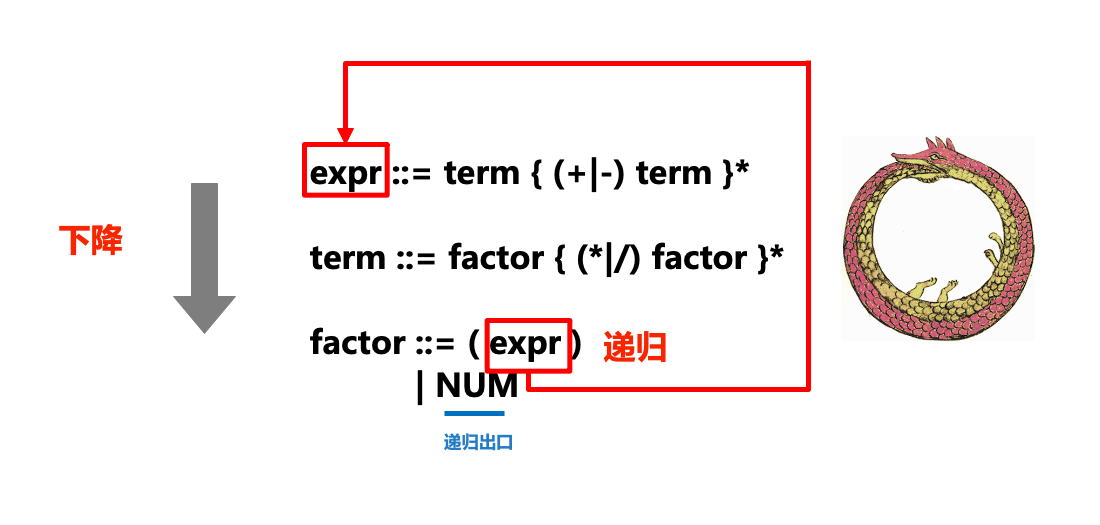# Python技法：实现简单的递归下降Parser

2022-04-30 17:06 585 查看

## 1. 算术运算表达式求值

`NUM+NUM*NUM`
（分词方法参见上一篇博文）。

```expr ::= expr + term
| expr - term
| term
term ::= term * factor
| term / factor
| factor
factor ::= (expr)
| NUM```

```expr ::= term { (+|-) term }*
term ::= factor { (*|/) factor }*
factor ::= (expr)
| NUM```

BNF和EBNF每一条规则(形如

`::=`

`{...}*`

`*````class ExpressionEvaluator():
...
def expr(self):
...
def term(self):
...
def factor(self):
...```

`expr`

`term`
`term`

`factor`
），则也就是递归下降中“下降”的部分。

`expr`

`term`
`term`

`factor`

`factor`

`expr`
，相当于一条衔尾蛇），这也就是递归下降中“递归”的部分。

`expr ::= term { (+|-) term }*`
），我们则通过
`while`

```import re
import collections

# 定义匹配token的模式
NUM = r'(?P<NUM>\d+)'  # \d表示匹配数字，+表示任意长度
PLUS = r'(?P<PLUS>\+)'  # 注意转义
MINUS = r'(?P<MINUS>-)'
TIMES = r'(?P<TIMES>\*)'  # 注意转义
DIVIDE = r'(?P<DIVIDE>/)'
LPAREN = r'(?P<LPAREN>\()'  # 注意转义
RPAREN = r'(?P<RPAREN>\))'  # 注意转义
WS = r'(?P<WS>\s+)'  # 别忘记空格，\s表示空格，+表示任意长度

master_pat = re.compile(
'|'.join([NUM, PLUS, MINUS, TIMES, DIVIDE, LPAREN, RPAREN, WS]))

# Tokenizer
Token = collections.namedtuple('Token', ['type', 'value'])

def generate_tokens(text):
scanner = master_pat.scanner(text)
for m in iter(scanner.match, None):
tok = Token(m.lastgroup, m.group())
if tok.type != 'WS':  # 过滤掉空格符
yield tok```

```class ExpressionEvaluator():
""" 递归下降的Parser实现，每个语法规则都对应一个方法，

"""

def parse(self, text):
""" 对外调用的接口 """
self.tokens = generate_tokens(text)
self.tok, self.next_tok = None, None  # 已匹配的最后一个token，下一个即将匹配的token
self._next()  # 转到下一个token
return self.expr()  # 开始递归

def _next(self):
""" 转到下一个token """
self.tok, self.next_tok = self.next_tok, next(self.tokens, None)

def _accept(self, tok_type):
""" 如果下一个token与tok_type匹配，则转到下一个token """
if self.next_tok and self.next_tok.type == tok_type:
self._next()
return True
else:
return False

def _except(self, tok_type):
""" 检查是否匹配，如果不匹配则抛出异常 """
if not self._accept(tok_type):
raise SyntaxError("Excepted"+tok_type)

# 接下来是语法规则，每个语法规则对应一个方法

def expr(self):
""" 对应规则： expression ::= term { ('+'|'-') term }* """
exprval = self.term() # 取第一项
while self._accept("PLUS") or self._accept("DIVIDE"): # 如果下一项是"+"或"-"
op = self.tok.type
# 再取下一项，即运算符右值
right = self.term()
if op == "PLUS":
exprval += right
elif op == "MINUS":
exprval -= right
return exprval

def term(self):
""" 对应规则： term ::= factor { ('*'|'/') factor }* """

termval = self.factor() # 取第一项
while self._accept("TIMES") or self._accept("DIVIDE"): # 如果下一项是"+"或"-"
op = self.tok.type
# 再取下一项，即运算符右值
right = self.factor()
if op == "TIMES":
termval *= right
elif op == "DIVIDE":
termval /= right
return termval

def factor(self):
""" 对应规则： factor ::= NUM | ( expr ) """
if self._accept("NUM"): # 递归出口
return int(self.tok.value)
elif self._accept("LPAREN"):
exprval = self.expr() # 继续递归下去求表达式值
self._except("RPAREN") # 别忘记检查是否有右括号，没有则抛出异常
return exprval
else:
raise SyntaxError("Expected NUMBER or LPAREN")```

```e = ExpressionEvaluator()
print(e.parse("2"))
print(e.parse("2+3"))
print(e.parse("2+3*4"))
print(e.parse("2+(3+4)*5"))```

```2
5
14
37```

`print(e.parse("2 + (3 + * 4)"))`

`SyntaxError`

`Expected NUMBER or LPAREN`
。 综上，可见我们的表达式求值算法运行正确。

## 2. 生成表达式树

```class ExpressionTreeBuilder(ExpressionEvaluator):
def expr(self):
""" 对应规则： expression ::= term { ('+'|'-') term }* """
exprval = self.term() # 取第一项
while self._accept("PLUS") or self._accept("DIVIDE"): # 如果下一项是"+"或"-"
op = self.tok.type
# 再取下一项，即运算符右值
right = self.term()
if op == "PLUS":
exprval = ('+', exprval, right)
elif op == "MINUS":
exprval -= ('-', exprval, right)
return exprval

def term(self):
""" 对应规则： term ::= factor { ('*'|'/') factor }* """

termval = self.factor() # 取第一项
while self._accept("TIMES") or self._accept("DIVIDE"): # 如果下一项是"+"或"-"
op = self.tok.type
# 再取下一项，即运算符右值
right = self.factor()
if op == "TIMES":
termval = ('*', termval, right)
elif op == "DIVIDE":
termval = ('/', termval, right)
return termval

def factor(self):
""" 对应规则： factor ::= NUM | ( expr ) """
if self._accept("NUM"): # 递归出口
return int(self.tok.value) # 字符串转整形
elif self._accept("LPAREN"):
exprval = self.expr() # 继续递归下去求表达式值
self._except("RPAREN") # 别忘记检查是否有右括号，没有则抛出异常
return exprval
else:
raise SyntaxError("Expected NUMBER or LPAREN")```

```print(e.parse("2+3"))
print(e.parse("2+3*4"))
print(e.parse("2+(3+4)*5"))
print(e.parse('2+3+4'))```

```('+', 2, 3)
('+', 2, ('*', 3, 4))
('+', 2, ('*', ('+', 3, 4), 5))
('+', ('+', 2, 3), 4)```

`Grammar`

## 左递归和运算符优先级陷阱

```items ::= items ',' item
| item```

```def items(self):
itemsval = self.items() # 取第一项，然而此处会无穷递归！
if itemsval and self._accept(','):
itemsval.append(self.item())
else:
itemsval = [self.item()]```

`self.items()`

```expr ::= factor { ('+'|'-'|'*'|'/') factor }*
factor ::= '(' expr ')'
| NUM```

`"3+4*5"`

`expr`
`term`